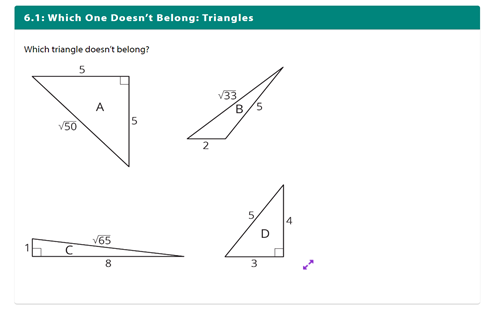Go to website

# Finding side lengths of triangles

A series of tasks to help students understand the basics of the Pythagorean theorem,which describes the relationship between the side lengths of right-angled triangles.

Year level(s) Year 9
Audience Student
Format Web page
Teaching strategies and pedagogical approaches Worked examples

## Curriculum alignment

Strand and focus Space
Topics Angles and geometric reasoning
AC: Mathematics (V9.0) content descriptions
AC9M9M03

Solve spatial problems, applying angle properties, scale, similarity, Pythagoras' theorem and trigonometry in right-angled triangles

Numeracy progression Understanding geometric properties (P7)
Understanding units of measurement (P10)
Proportional thinking (P7)